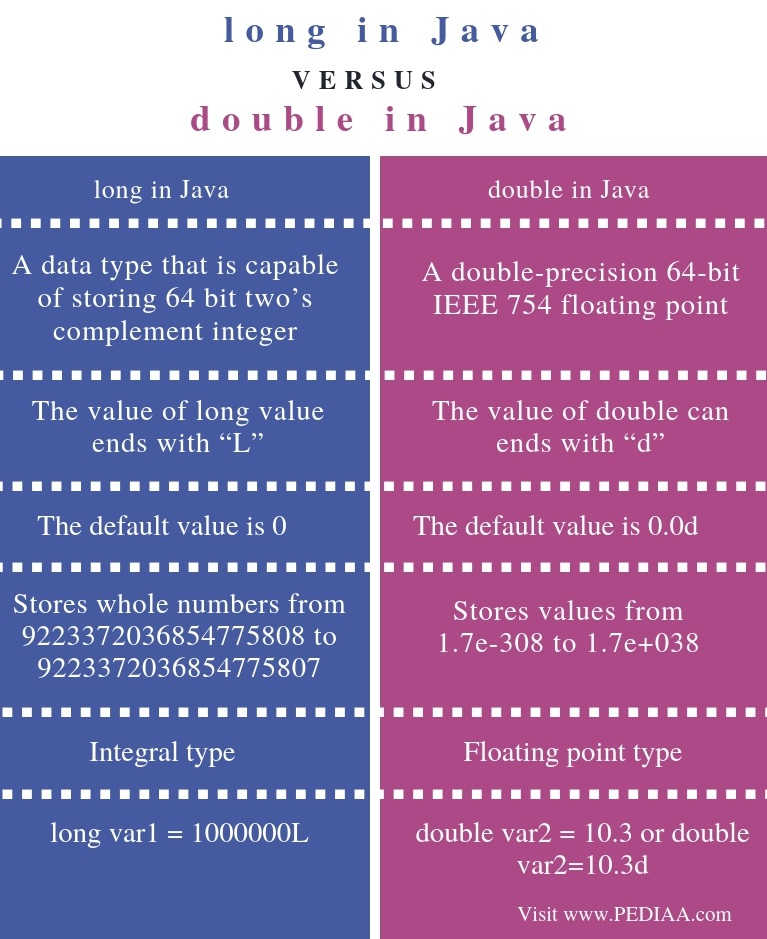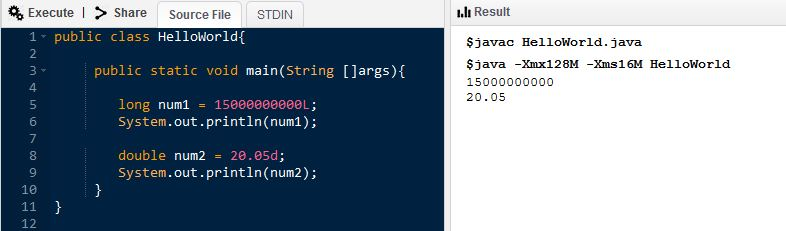# What is the Difference Between long and double in Java

The main difference between long and double in Java is that long is a data type that stores 64 bit two’s complement integer while double is a data type that stores double prevision 64 bit IEEE 754 floating point.

In programming, it is necessary to store data. A variable is a name given to a memory location that stores data. Each variable has a data type which defines the data type the variable can store. In other words, the data type also explains the amount of memory allocated to store that data.  Two primitive data types in Java programming languages are long and double. In Java, both these data types require 8 bytes to store data.

### Key Areas Covered

1. What is long in Java
– Definition, Functionality
2. What is double in Java
– Definition, Functionality
3. Difference Between long and double in Java
– Comparison of Key Differences

### Key Terms

double, long, Java## What is long in Java

“long” is a data type that can store whole numbers from 9223372036854775808 to 9223372036854775807. Another common data type is int. It is used to store integer values. When the int data type is not large enough to store a value, the programmer can use long data type. The value of long ends with letter “L”. Furthermore, the default value of long is 0.

## What is double in Java

“double” is a data type that stores floating point numbers. It is similar to a float data type. Unlike, a float which stores 32 bit IEEE 745 floating point numbers, double stores 64-but IEEE 754 floating point numbers. It can store values from 1.7e-308 to 1.7e+038. The double value ends with the letter “d”. It is also possible to write the value without writing d. Furthermore, the default value of double is 0.0d.Figure 1: Java program with long and double data types

Above is a simple program with long and double variables. The num1 variable is type long. Similarly, num2 variable is type double. The system.out.println statements display the corresponding values on the console.

## Difference Between long and double in Java

### Definition

long is a data type that is capable of storing 64 bit two’s complement integer. In contrast, double data type is a double-precision 64-bit IEEE 754 floating point. Thus, this is the main difference between long and double in Java.

### Ending the value

Also, another difference between long and double in Java is that the value of long value ends with “L” while the value of double ends with “d”.

### Default value

The default value of long is 0 whereas the default value of double is 0.0d.

### Values range

Furthermore, the data type long stores whole numbers from 9223372036854775808 to 9223372036854775807. On the other hand, double stores values from 1.7e-308 to 1.7e+038.

### Type

Moreover, one other difference between long and double in Java is that long is an integral type while double is a floating point type.

### Example

Additionally, long var1 = 1000000L is an example for long data type while double var2 = 10.3 or double var2=10.3d is an example for double data type.

### Conclusion

In conclusion, long and double are two primitive data types available in Java programming language. Programmer can use a data type according to the storing data. The main difference between long and double in Java is that long is a data type that stores 64 bit two’s complement integer while double is a data type that stores double prevision 64 bit IEEE 754 floating point. In brief, long is an integral type whereas double is a floating point type.

##### Reference:

1.Java Data Types, Available here.
2.“Data Types in Java.” GeeksforGeeks, 7 Sept. 2018, Available here.
3.“Java Data Types – Javatpoint.” Www.javatpoint.com, Available here.